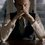# Calculus Challenge 8!

$\displaystyle R^-_n = \frac{2}{\pi} \int_{0}^{\pi/2}{{(\theta^2 + \ln^2(\cos \theta))}^{-{2}^{-n-1}} \sqrt{\frac{1}{2} + \frac{1}{2}\sqrt{\frac{1}{2} + \cdots + \frac{1}{2}\sqrt{\frac{\ln^2(\cos \theta)}{\theta^2 + \ln^2(\cos \theta)}}}} \ d\theta} = {(\ln 2)}^{-{2}^{-n}}$ $\displaystyle R^+_n = \frac{2}{\pi} \int_{0}^{\pi/2}{{(\theta^2 + \ln^2(\cos \theta))}^{{2}^{-n-1}} \sqrt{\frac{1}{2} + \frac{1}{2}\sqrt{\frac{1}{2} + \cdots + \frac{1}{2}\sqrt{\frac{\ln^2(\cos \theta)}{\theta^2 + \ln^2(\cos \theta)}}}} \ d\theta} = {(\ln 2)}^{{2}^{-n}}$

Prove the above 2 AMAZING integrals (I must add that adjective, pardon me!)Note by Kartik Sharma
5 years, 7 months ago

This discussion board is a place to discuss our Daily Challenges and the math and science related to those challenges. Explanations are more than just a solution — they should explain the steps and thinking strategies that you used to obtain the solution. Comments should further the discussion of math and science.

When posting on Brilliant:

• Use the emojis to react to an explanation, whether you're congratulating a job well done , or just really confused .
• Ask specific questions about the challenge or the steps in somebody's explanation. Well-posed questions can add a lot to the discussion, but posting "I don't understand!" doesn't help anyone.
• Try to contribute something new to the discussion, whether it is an extension, generalization or other idea related to the challenge.

MarkdownAppears as
*italics* or _italics_ italics
**bold** or __bold__ bold
- bulleted- list
• bulleted
• list
1. numbered2. list
1. numbered
2. list
Note: you must add a full line of space before and after lists for them to show up correctly
paragraph 1paragraph 2

paragraph 1

paragraph 2

[example link](https://brilliant.org)example link
> This is a quote
This is a quote
    # I indented these lines
# 4 spaces, and now they show
# up as a code block.

print "hello world"
# I indented these lines
# 4 spaces, and now they show
# up as a code block.

print "hello world"
MathAppears as
Remember to wrap math in $$ ... $$ or $ ... $ to ensure proper formatting.
2 \times 3 $2 \times 3$
2^{34} $2^{34}$
a_{i-1} $a_{i-1}$
\frac{2}{3} $\frac{2}{3}$
\sqrt{2} $\sqrt{2}$
\sum_{i=1}^3 $\sum_{i=1}^3$
\sin \theta $\sin \theta$
\boxed{123} $\boxed{123}$

Sort by:

asd

- 4 years, 6 months ago

- 5 years, 7 months ago

Can u post the solution?

- 5 years, 7 months ago

Hint: The infinite surds reminds you of what? $\cos(x) = \sqrt{\frac{1}{2} + \frac{\cos(2x)}{2}}$

- 5 years, 7 months ago

We can simplify the integral to $\displaystyle R^-_n = \frac{2}{\pi} \int_{0}^{\pi/2} {(\theta^2 + \ln^2(\cos \theta))}^{-{2}^{-n-1}} \cdot \cos \left(\dfrac{1}{2^n} \cos^{-1} \left(\sqrt{ \frac{\ln^2(\cos \theta)}{\theta^2 + \ln^2(\cos \theta)} }\right) \right)$ and

$\displaystyle R^+_n = \frac{2}{\pi} \int_{0}^{\pi/2} {(\theta^2 + \ln^2(\cos \theta))}^{{2}^{-n-1}} \cdot \cos \left(\dfrac{1}{2^n} \cos^{-1} \left(\sqrt{ \frac{\ln^2(\cos \theta)}{\theta^2 + \ln^2(\cos \theta)} }\right) \right)$

but what to do after that?

- 5 years, 7 months ago